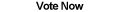Home Articles Components Code Snippets Links

C# Code Snippets# C# Distance Formula

Visual C# Kicks

The C# function returns the distance between two points using Pythagorean Theorem.

Platform: .NET Framework 2.0

```private static double GetDistance(PointF point1, PointF point2)
{
//pythagorean theorem c^2 = a^2 + b^2
//thus c = square root(a^2 + b^2)
double a = (double)(point2.X - point1.X);
double b = (double)(point2.Y - point1.Y);

return Math.Sqrt(a * a + b * b);
}```

Platform: .NET Framework 3.5
Extension verison

```public static class PointFunctions
{
public static double DistanceTo(this Point point1, Point point2)
{
var a = (double)(point2.X - point1.X);
var b = (double)(point2.Y - point1.Y);

return Math.Sqrt(a * a + b * b);
}
}```

Back to C# Code Snippet List